# 磁珠的阻抗曲线，想多了....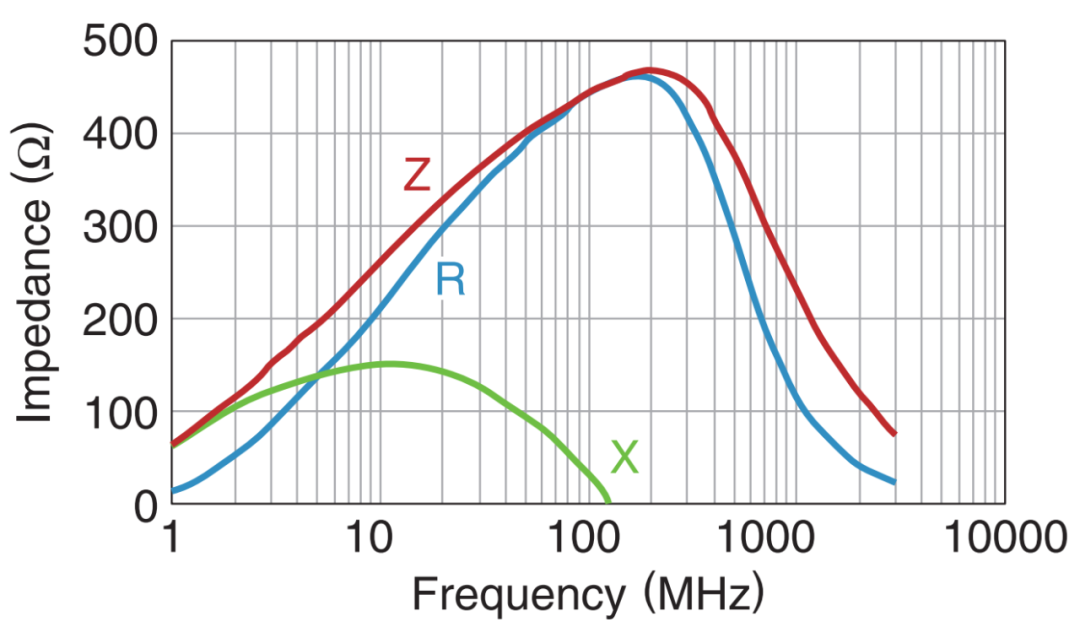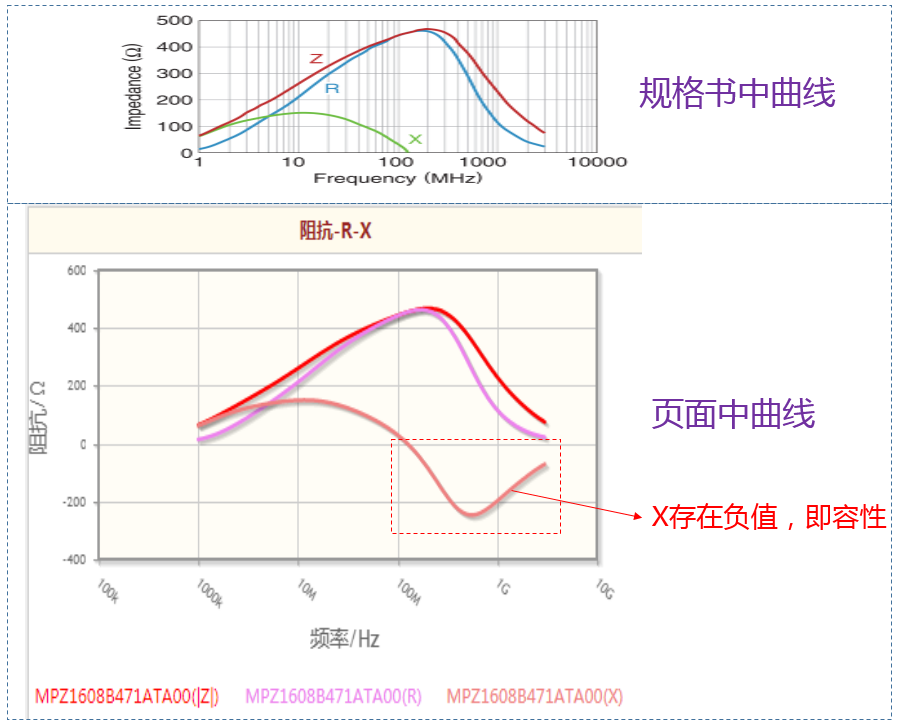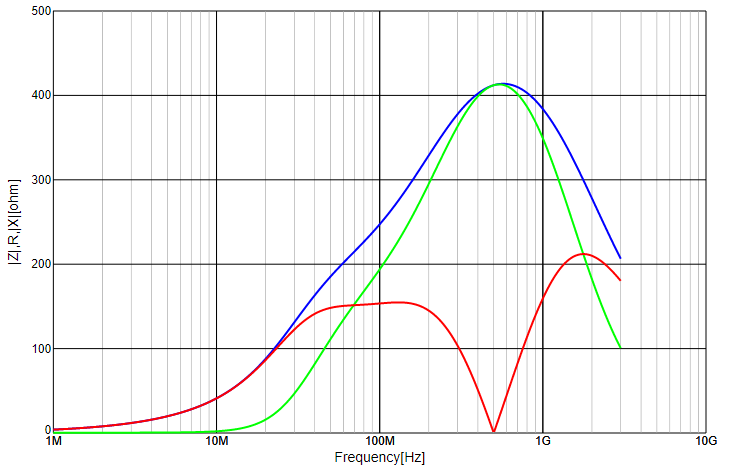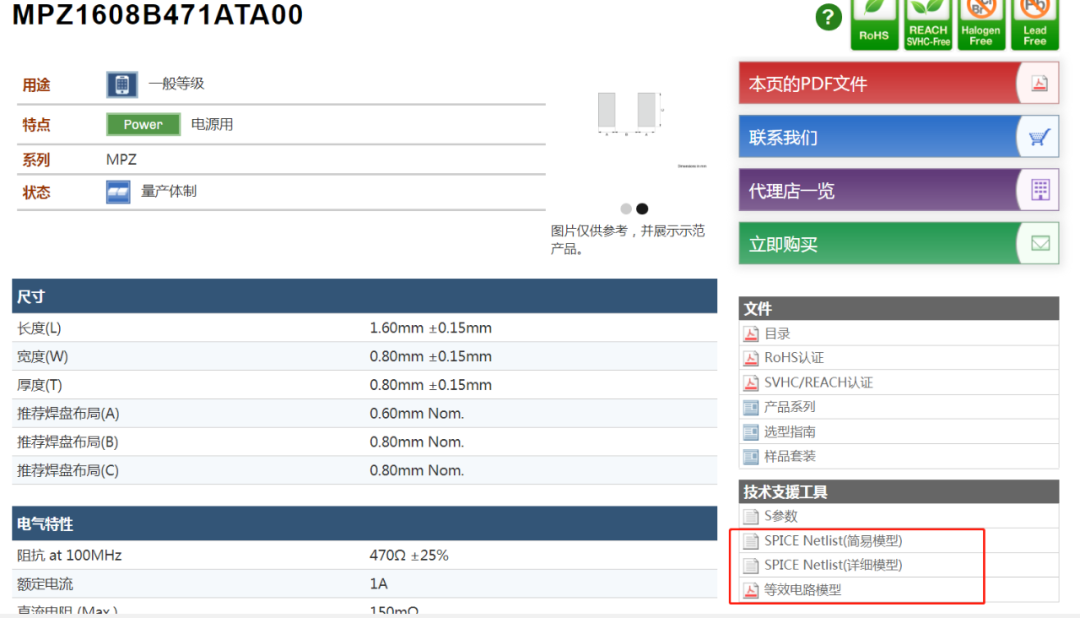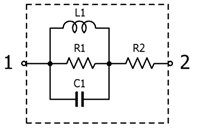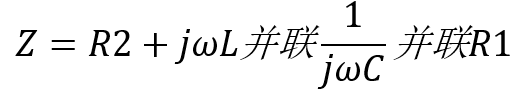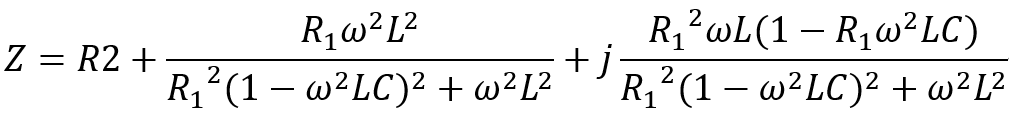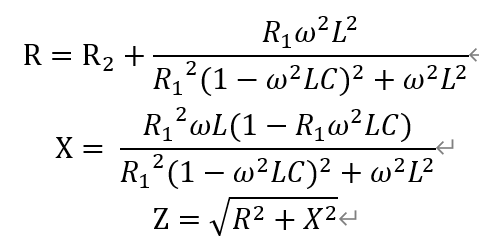MPZ1608B471ATA00的参数从pdf文档中知道，R1=470Ω，L1=8.6uH，C1=0.2583pF，R2=0.110Ω。

``````C1=0.0000000000002583; %0.2583pF
L1=0.0000086;        %8.6uH
R1=470;            %470Ω
R2=0.11;             %DCR:0.110Ω
f=[1000000:100000:10000000000];  %1Mhz-1Ghz
w=(f.*pi*2);
R=R2+(w.^2.*R1*L1^2)./((1-w.^2*L1*C1).^2*R1^2+w.^2*L1^2);
X=w.*L1*R1^2.*(1-w.^2*L1*C1)./((1-w.^2*L1*C1).^2*R1^2+w.^2*L1^2);
Z=(R.^2+X.^2).^0.5;
figure;
semilogx(f,Z,'g',f,X,'r',f,R,'b');
legend('Z','X','R');
grid on;
set(gca, 'XTickLabel' ,{'1M','10M','100M','1G','10G'});
xlabel('频率'), ylabel('|阻抗Ω|');
title '磁珠阻抗-频率曲线';
axis([1000000 10000000000 0 500]);``````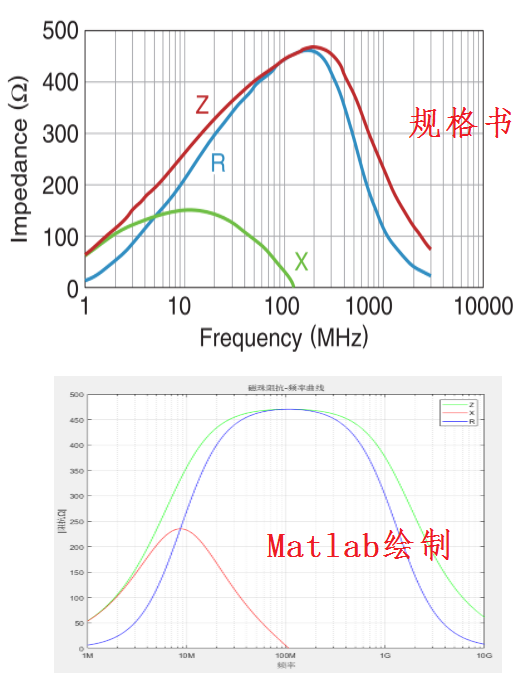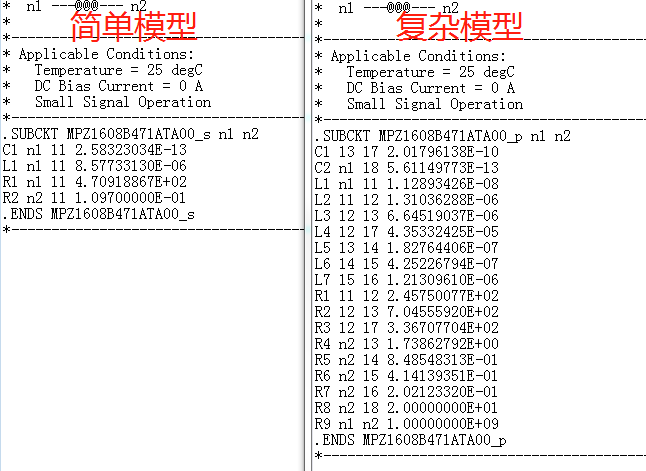0/200